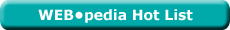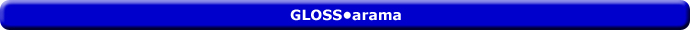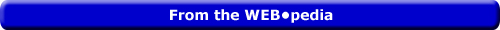Saturday  June 25, 2022
 AmosWEB means Economics with a Touch of Whimsy!YTM: The common abbreviation for yield to maturity, which is the annual rate of return on a financial asset that is held until maturity. Yield to maturity depends on both the coupon rate and the face or par value paid at maturity. If the selling price of a financial asset is equal to its par value, then the yield to maturity is equal to the current yield and the coupon rate. However, if the asset is selling at a discount, then the yield to maturity exceeds the current yield, which is greater than the coupon rate. And if the asset is selling at a premium, then the yield to maturity is less than the current yield, which is below than the coupon rate.Most Viewed (Number) Visit the WEB*pediaPERFECT COMPETITION, MARGINAL ANALYSIS: A perfectly competitive firm produces the profit-maximizing quantity of output that equates marginal revenue and marginal cost. This marginal approach is one of three methods that used to determine the profit-maximizing quantity of output. The other two methods involve the direct analysis of economic profit or a comparison of total revenue and total cost.Recommended Citation:

PERFECT COMPETITION, MARGINAL ANALYSIS, AmosWEB GLOSS*arama, http://www.AmosWEB.com, AmosWEB LLC, 2000-2022. [Accessed: June 25, 2022].

AmosWEB Encyclonomic WEB*pedia:

Additional information on this term can be found at:

WEB*pedia: perfect competition, marginal analysis

Search Again?LOSS MINIMIZATION RULE

A rule stating that a firm minimizes economic loss by producing output in the short run that equates marginal revenue and marginal cost if price is less than average total cost but greater than average variable cost. This is one of three short-run production alternatives facing a firm. The other two are profit maximization (if price exceeds average total cost) and shutdown (if price is less than average variable cost).BLUE PLACIDOLA[What's This?] Today, you are likely to spend a great deal of time watching the shopping channel trying to buy either a birthday gift for your father that doesn't look like every other birthday gift for your father or a green fountain pen. Be on the lookout for poorly written technical manuals.Your Complete ScopeThe portion of aggregate output U.S. citizens pay in taxes (30%) is less than the other six leading industrialized nations -- Britain, Canada, France, Germany, Italy, or Japan."It is not fair to ask of others what you are unwilling to do yourself. "-- Eleanor Roosevelt, diplomat, activistFACFederal Advisory CouncilA PEDestrian's Guide Xtra CreditTell us what you think about AmosWEB. Like what you see? Have suggestions for improvements? Let us know. Click the User Feedback link.| | | | | | | | | | |
| | | |

Thanks for visiting AmosWEB